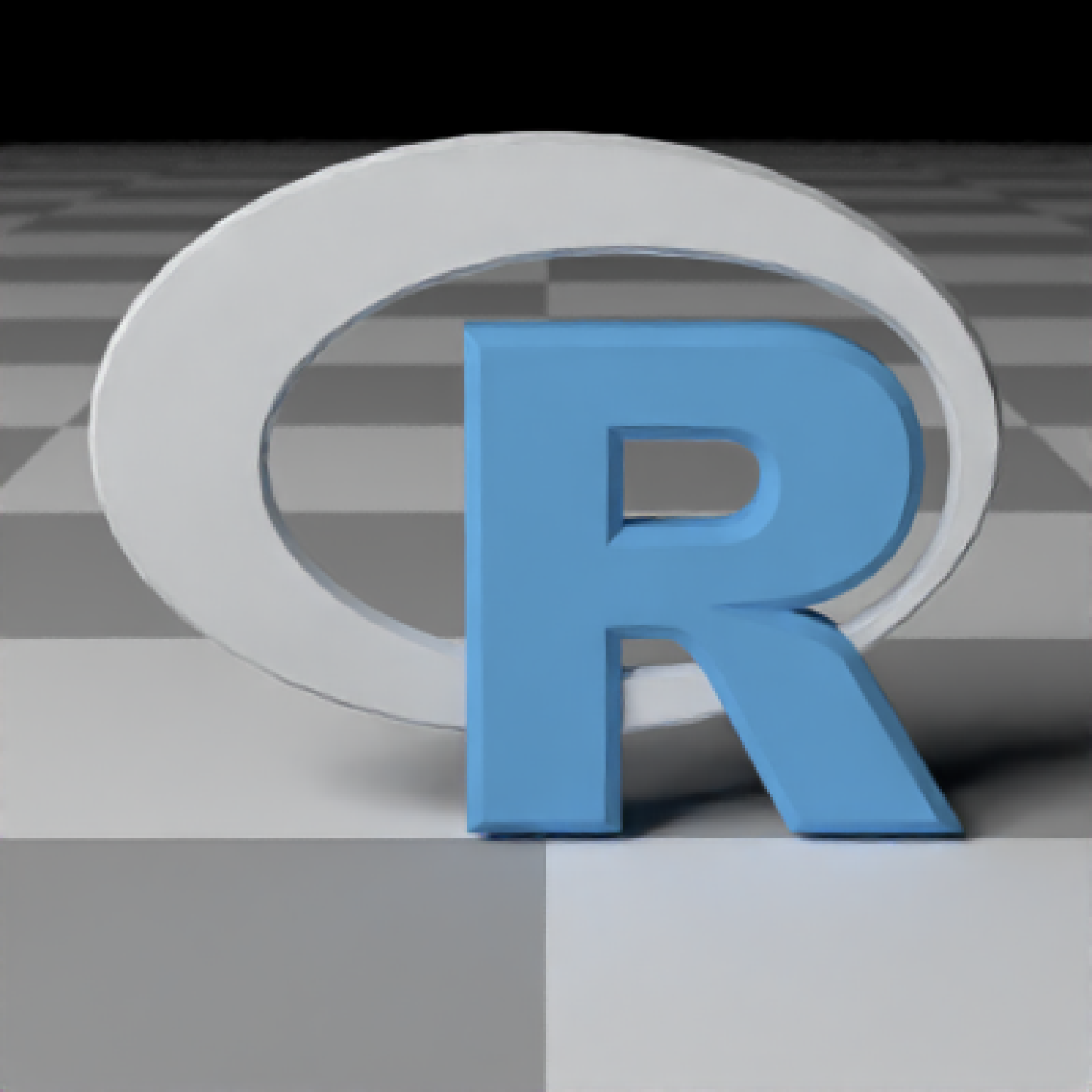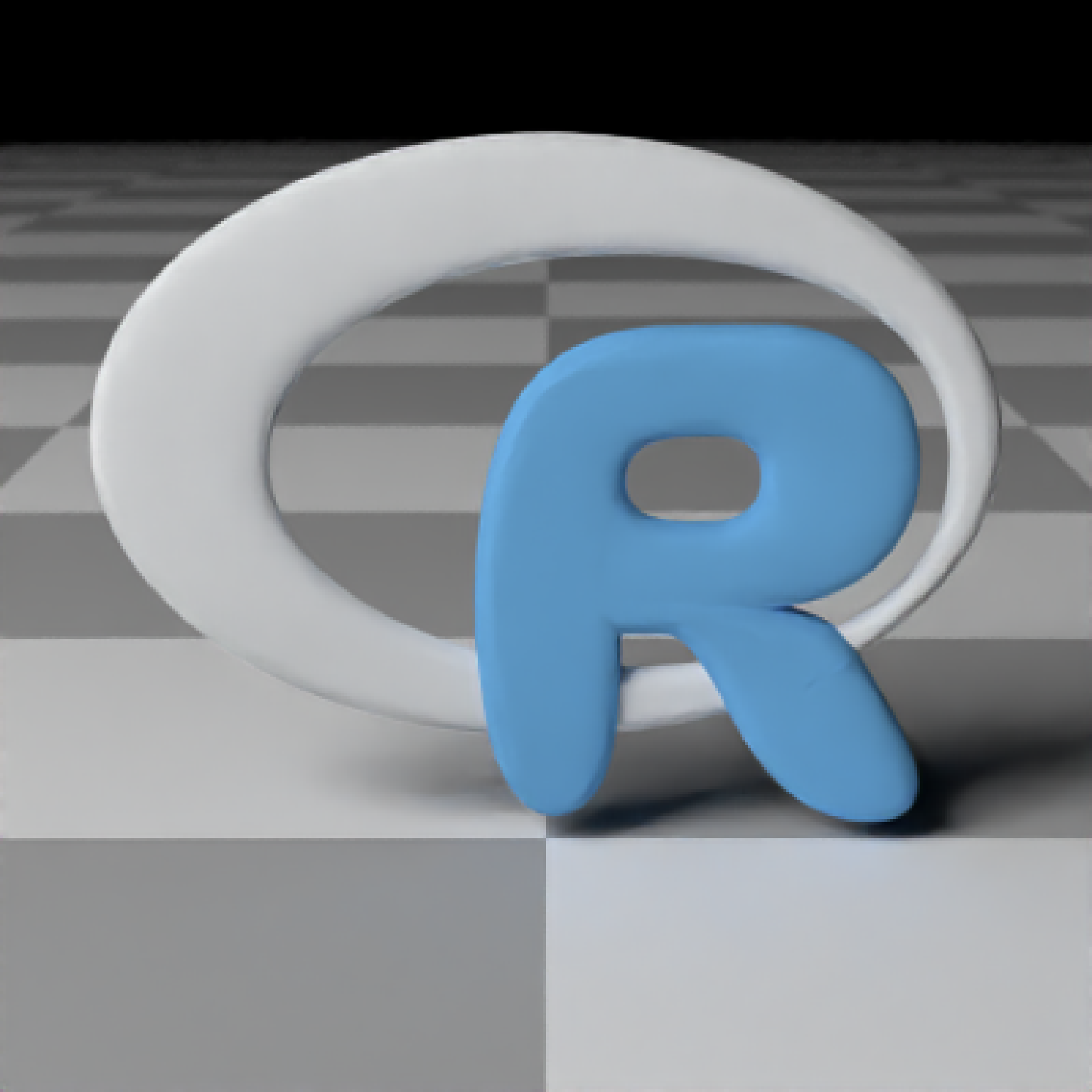Load an obj file via a filepath. Currently only supports the diffuse texture with the texture argument. Note: light importance sampling currently not supported for this shape.

obj_model(
filename,
x = 0,
y = 0,
z = 0,
scale_obj = 1,
vertex_colors = FALSE,
calculate_consistent_normals = TRUE,
importance_sample_lights = TRUE,
material = diffuse(),
angle = c(0, 0, 0),
order_rotation = c(1, 2, 3),
flipped = FALSE,
scale = c(1, 1, 1)
)

## Arguments

filename

Filename and path to the obj file. Can also be a txt file, if it's in the correct obj internally.

x

Default 0. x-coordinate to offset the model.

y

Default 0. y-coordinate to offset the model.

z

Default 0. z-coordinate to offset the model.

scale_obj

Default 1. Amount to scale the model. Use this to scale the object up or down on all axes, as it is more robust to numerical precision errors than the generic scale option.

Default TRUE. Whether to load the obj file material (MTL file). If material for faces aren't specified, the default material will be used (specified by the user in material).

Default TRUE. If load_material = TRUE, whether to load textures in the MTL file (versus just using the colors specified for each material).

Default TRUE. Whether to load the vertex normals if they exist in the OBJ file.

vertex_colors

Default FALSE. Set to TRUE if the OBJ file has vertex colors to apply them to the model.

calculate_consistent_normals

Default TRUE. Whether to calculate consistent vertex normals to prevent energy loss at edges.

importance_sample_lights

Default TRUE. Whether to importance sample lights specified in the OBJ material (objects with a non-zero Ke MTL material).

material

Default diffuse.The material, called from one of the material functions diffuse, metal, or dielectric.

angle

Default c(0, 0, 0). Angle of rotation around the x, y, and z axes, applied in the order specified in order_rotation.

order_rotation

Default c(1, 2, 3). The order to apply the rotations, referring to "x", "y", and "z".

flipped

Default FALSE. Whether to flip the normals.

scale

Default c(1, 1, 1). Scale transformation in the x, y, and z directions. If this is a single value, number, the object will be scaled uniformly. Note: emissive objects may not currently function correctly when scaled.

## Value

Single row of a tibble describing the obj model in the scene.

## Examples

#Load the included example R object file, by calling the r_obj() function. This
#returns the local file path to the r.txt obj file. The file extension is "txt"
#due to package constraints, but the file contents are identical and it does not
#affect the function.

if(run_documentation()) {
generate_ground(material = diffuse(checkercolor = "grey50")) %>%
add_object(obj_model(y = -0.8, filename = r_obj(),
material = microfacet(color = "gold", roughness = 0.05))) %>%
add_object(obj_model(x = 1.8, y = -0.8, filename = r_obj(),
material = diffuse(color = "dodgerblue"))) %>%
add_object(obj_model(x = -1.8, y = -0.8, filename = r_obj() ,
material = dielectric(attenuation = c(1,0.3,1)*2))) %>%
add_object(sphere(z = 20, x = 20, y = 20, radius = 10,
material = light(intensity = 10))) %>%
render_scene(parallel = TRUE, samples = 128, aperture = 0.05,
fov = 32, lookfrom = c(0, 2, 10))

}#Use scale_obj to make objects bigger--this is more robust than the generic scale argument.
if(run_documentation()) {
generate_ground(material = diffuse(checkercolor = "grey50")) %>%
add_object(obj_model(y = -0.8, filename = r_obj(), scale_obj = 2,
material = diffuse(noise = TRUE, noiseintensity = 10,noisephase=45))) %>%
add_object(sphere(z = 20, x = 20, y = 20, radius = 10,
material = light(intensity = 10))) %>%
render_scene(parallel = TRUE, samples = 128, ambient = TRUE,
backgroundhigh="blue", backgroundlow="red",
aperture = 0.05, fov = 32, lookfrom = c(0, 2, 10),
lookat = c(0,1,0))
}